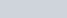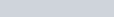The Newton-Raphson method is another numerical method for solving equations of the formThis is best illustrated by the example below which is covered in the video.

Example:

1. Find an approximation to the root ofusing the Newton-Raphson method to two decimal places, given that the root lies between 0 and 1.
Newton-Raphson method - Maths Made Easy - youtube Video